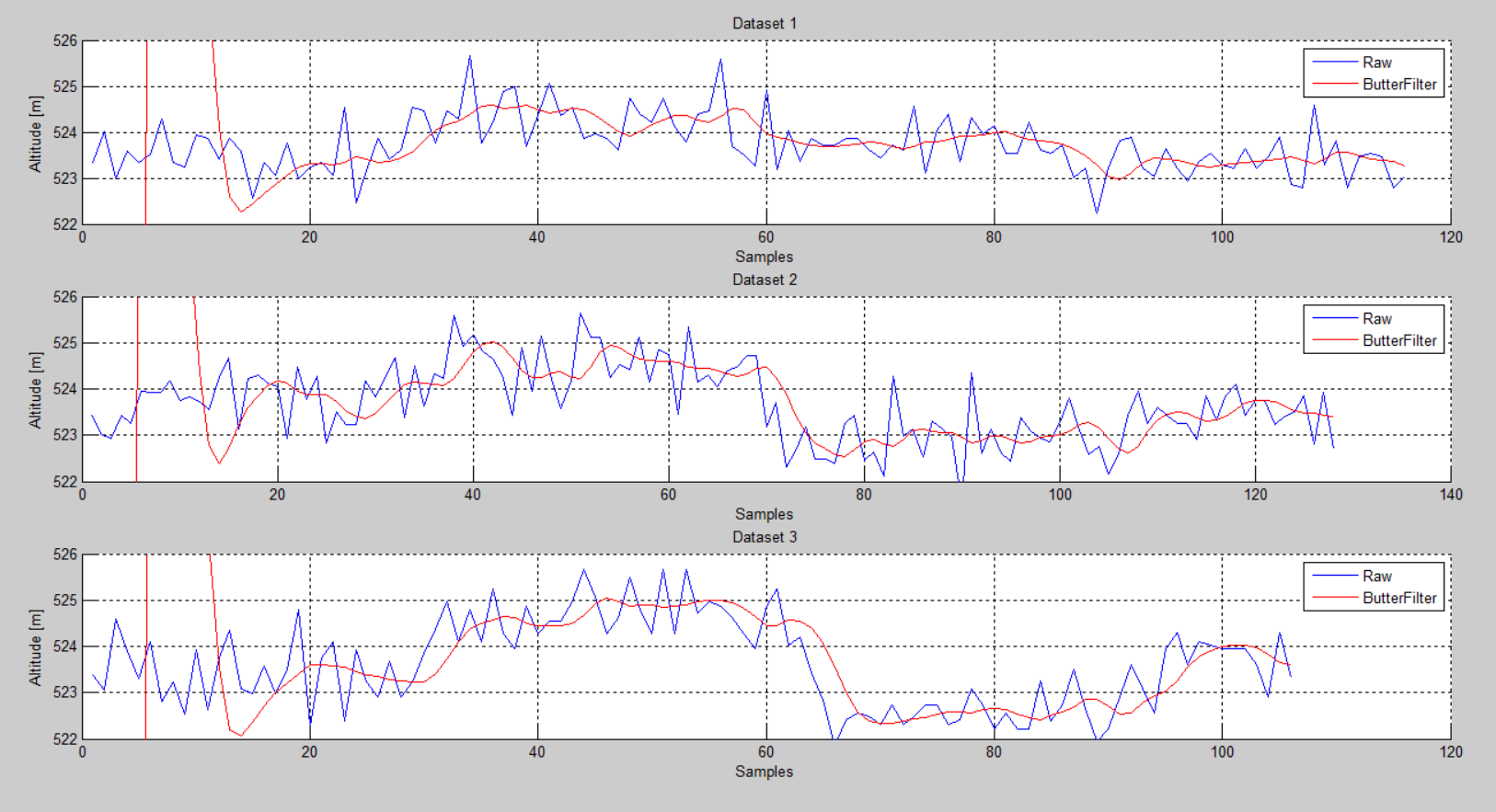02 Dec

#### Blimp Altitude Filtering Algorithm (Second Order)

This past week, I focused on improving upon the altitude filtering algorithms I had tested previously for the blimps on FORAY. The previous two algorithms I had implemented (both in arduino and in MATLAB) were a moving average filter and an exponential filter (both first-order filters). Both introduced a notable amount of lag into the signal and as such I idecided to investigate implementing a higher-order filter.

In MATLAB, I created a second-order Butterworth filter (low-pass) and used it to filter the data. The results for three datasets can be seen below.Comparing this to the previous two filters, it can be seen that the filter has a faster response time (less lag), while still filtering out the higher frequency noise (see below).The next step is to implement this algorithm for use in arduino and to test for real-time data collection.